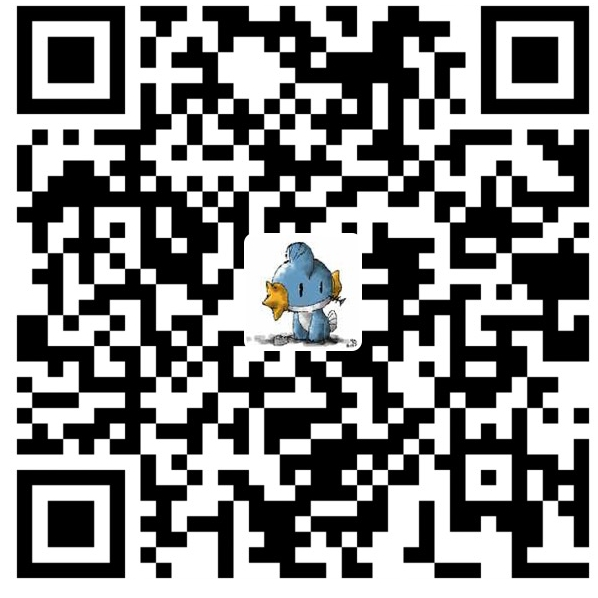# VBS基础教程第四篇

dim sum
sum=0 '初始化变量
sum=sum + int(inputbox("请输入交易额"))
'sum=sum+x这种形式是把本身的值取出来, 进行一次运算, 再放回本身, 这种方法很有用处
'这里使用了函数嵌套, 把inputbox的返回值直接传给int函数, 转化成整数, 下同
sum=sum + int(inputbox("请输入交易额"))
sum=sum + int(inputbox("请输入交易额"))
sum=sum + int(inputbox("请输入交易额"))
sum=sum + int(inputbox("请输入交易额"))
msgbox(sum)

do...loop的结构看上去非常简单, 就是:do...loop, 仅此而已, 这个结构不断执行do和loop之间的语句(学名叫:循环体),永不停止. 举个例子来说:

do
msgbox("这个信息会不断重复出现, 要停止程序请使用任务管理器(Ctrl+Alt+Del)中止wscript进程")
loop

dim a '注意:常量不需要在dim里面声明,否则会引发错误
const pass="123456" '这是一个字符串 请用""包裹起来. 设定密码为常量, 不可变更
do
a=inputbox("请输入密码")
if a=pass then
msgbox("密码校验成功")
exit do
end if
loop

dim a,ctr
ctr=0 '设置计数器
const pass="pas123_" '上面的那个是弱密码, 这次改的强一点
do
if ctr=3 then
msgbox("已经达到认证上限, 认证程序关闭")
exit do
else
a=inputbox("请输入密码")
if a=pass then
msgbox("认证成功")
msgbox("(你可以在这里加一段成功后得到的信息)")
exit do
else
ctr=ctr+1 '如果密码出错就增加一次错误认证计数
msgbox("认证出错, 请检查密码")
end if
end if
loop

dim a,ctr
ctr=0
const pass="pas123_"
do while ctr<3
a=inputbox("请输入密码")
if a=pass then
msgbox("认证成功")
msgbox("(你可以在这里加一段成功后得到的信息)")
exit do
else
ctr=ctr+1 '如果密码出错就增加一次错误认证计数
msgbox("认证出错, 请检查密码")
end if
loop

dim a,ctr
ctr=0
const pass="pas123_"
do
a=inputbox("请输入密码")
if a=pass then
msgbox("认证成功")
msgbox("(你可以在这里加一段成功后得到的信息)")
exit do
else
ctr=ctr+1 '如果密码出错就增加一次错误认证计数
msgbox("认证出错, 请检查密码")
end if
loop while ctr<3

ok, 我们来看另外一种循环结构,for....next, 这种循环结构是基于计数的, 也是在编程中最常见到的循环结构.

dim i
for i=0 to 5
msgbox(i)
next

dim i
i=0
do while i<5
msgbox(i)
i=i+1 '因为do不能自动计数, 必须手动加
loop

dim i,j
for i=1 to 9
for i=1 to 9
str=str & i * j & " " '&是和并字符串的符号
next '每个next对应一个for
next

dim i,j
for i=1 to 9
for i=1 to 9
str=str & i * j & " "
next '每个next对应一个for
str=str & vbCrlf 'vbCrlf相当于键盘上的回车键,因为你不能在键盘上输入,所以系统定义了一个默认的常量
next

1) do..loop和exit do的用法

2) while当表达式true的时候执行循环体,until反之

3) for...next是计数循环, 每次执行计数器递加

4) 嵌套循环的作用和写法

4.5) &用于连接字符串

5) vbCrLf相当于键盘上的回车键

1) 在我国的数学经典著作"九章算术"中有这样一道题:百钱买百鸡, 公鸡5钱一只, 母鸡3钱一只, 小鸡1钱2只(这个数据我是参考一本编程书的, 但我记得是公3,母1,小1钱3只? 不管了, 就按照书上得来吧)求得是能有多少种办法买这些鸡. 如果看不懂的话我用大白话说说:有人要去买鸡, 用100块钱正好买了100只鸡, 价格如下:公:5\$, 母:3\$, 小:1\$ for 2, 让你求一共多少种卖法(公母小怎么搭配). 请用循环解决这个问题.

ps:今天要去移民局办事, 所以文章写的很仓促. 大部分代码都没有实验, 请大家帮我找错. 另外, 上次的作业大家在论坛应该看到了, 我就不重复答案了, 不知道大家喜欢做这种题, 还是以前那种比较简单的?微信赞赏支付宝赞赏